# 【目标检测-YOLO】YOLOv5-v5.0-yolov5s网络架构详解（第一篇）

## 1. 前言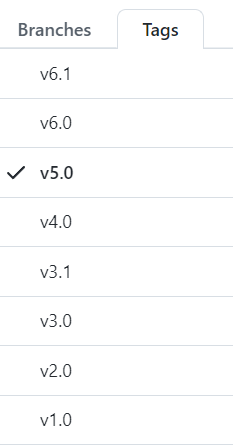## 2.修改过程

Releases · ultralytics/yolov5 · GitHub 找到 v5.0 中的 Assets 中的 yolov5s.pt 并下载该权重。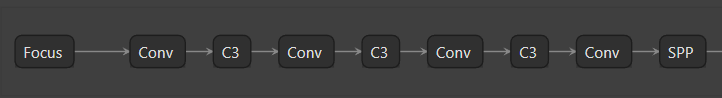为了看到更多细节，使用Netron 对 yolov5s.onnx 可视化：

python models/export.py –weights ./weights/yolov5s.pt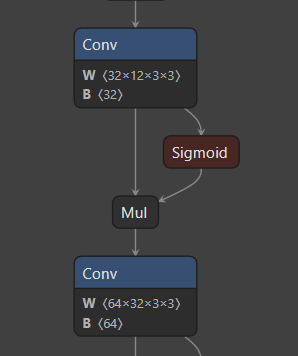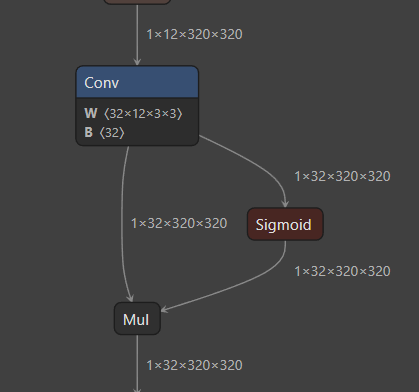显然该图没有显示输出输出的尺寸，为此我们修改 export.py 代码，并重新导出onnx，显示为上图右。

        from onnx import shape_inference
# Checks
onnx_model = onnx.load(f)  # load onnx model
onnx.save(shape_inference.infer_shapes(onnx_model), f)

# # Checks
# onnx_model = onnx.load(f)  # load onnx model

B其实就是 bias，但是 Conv2d + BN + SiLU 中 conv 是没有 bias的，那这个bias 怎么来的？其实这是因为在 fuse_conv_and_bn(conv, bn) 函数中：

    # prepare spatial bias
b_conv = torch.zeros(conv.weight.size(0), device=conv.weight.device) if conv.bias is None else conv.bias
b_bn = bn.bias - bn.weight.mul(bn.running_mean).div(torch.sqrt(bn.running_var + bn.eps))
fusedconv.bias.copy_(torch.mm(w_bn, b_conv.reshape(-1, 1)).reshape(-1) + b_bn)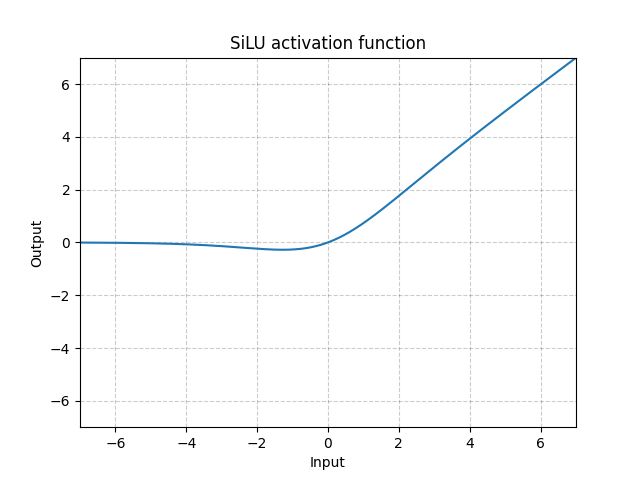def fuse(self):  # fuse model Conv2d() + BatchNorm2d() layers
print('Fusing layers... ')
# for m in self.model.modules():
#     if type(m) is Conv and hasattr(m, 'bn'):
#         m.conv = fuse_conv_and_bn(m.conv, m.bn)  # update conv
#         delattr(m, 'bn')  # remove batchnorm
#         m.forward = m.fuseforward  # update forward
# self.info()
return self

        torch.onnx.export(model, img, f, verbose=False, opset_version=12,
training=torch.onnx.TrainingMode.TRAINING,
input_names=['images'],
output_names=['classes', 'boxes'] if y is None else ['output'],
dynamic_axes={'images': {0: 'batch', 2: 'height', 3: 'width'},  # size(1,3,640,640)
'output': {0: 'batch', 2: 'y', 3: 'x'}} if opt.dynamic else None)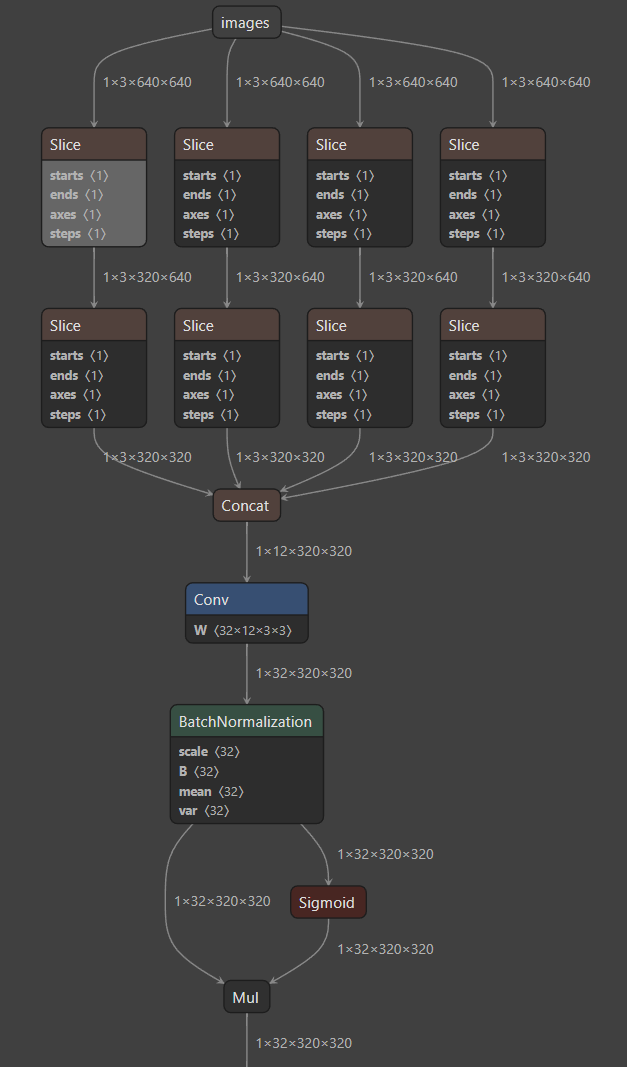当然，除了以上方式，当我们关闭fuse()后，也可以直接使用 Netron 对 导出的 weights/yolov5s.torchscript.pt 可视化，一样可以看到 BN。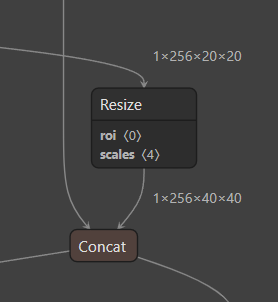出现下图中的原因是：

• 对于任何用到shape、size返回值的参数时，例如：tensor.view(tensor.size(0), -1)这类操作，避免直接使用tensor.size的返回值，而是加上int转换，tensor.view(int(tensor.size(0)), -1)。为了避免pytorch 导出 onnx 时候，对size 进行跟踪，跟踪时候会生成gather、shape的节点。
•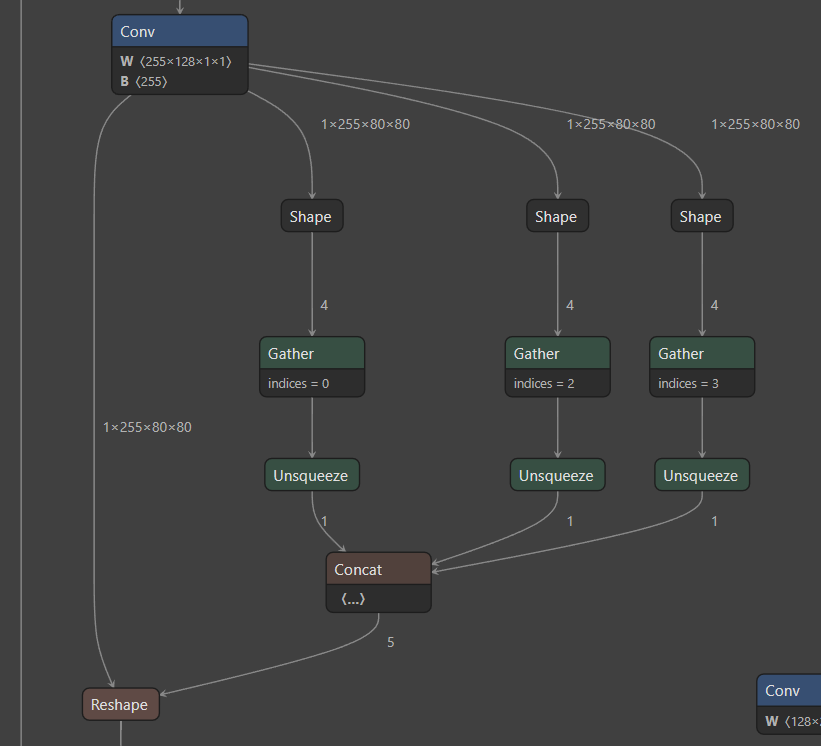因此修改代码：models/yolo.py

            # bs, _, ny, nx = x[i].shape  # x(bs,255,20,20) to x(bs,3,20,20,85)

bs, _, ny, nx = map(int, x[i].shape)

重新导出 onnx，如下图，看起来清爽多了。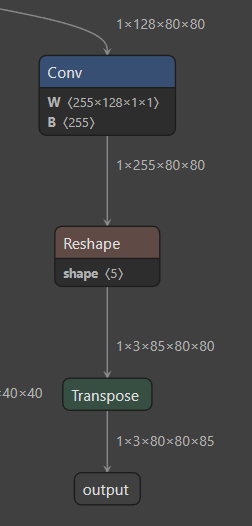## 3. 最终成图

使用PPT作图：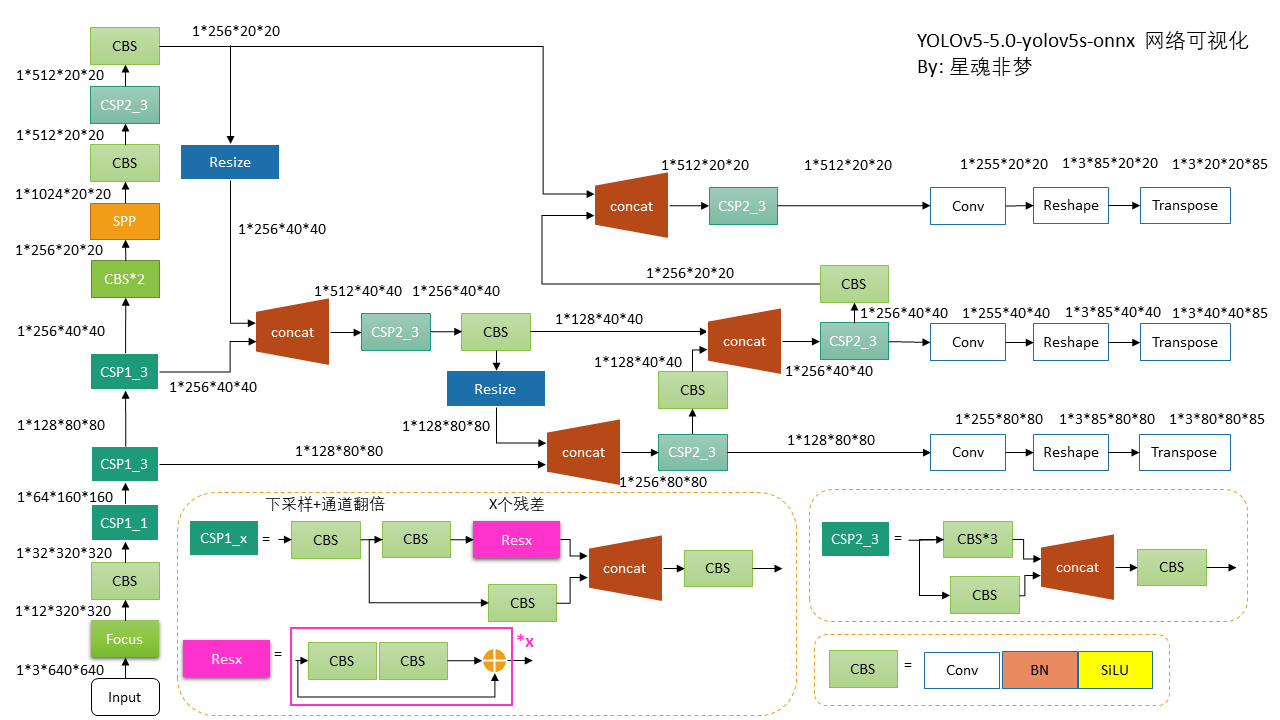注意：在代码中

• 图中 Resize 为 nn.Upsample_nearest
• Reshape 为 view，Transpose 为 permute

• 以上所有的带 BN的 conv 都是没有 bias的， 只有最后的Conv 带 bias

• 来源：理心炼丹